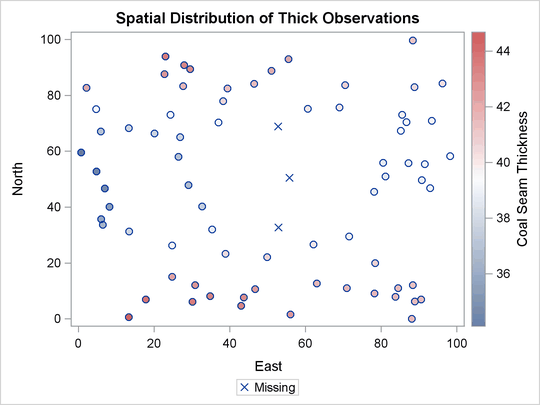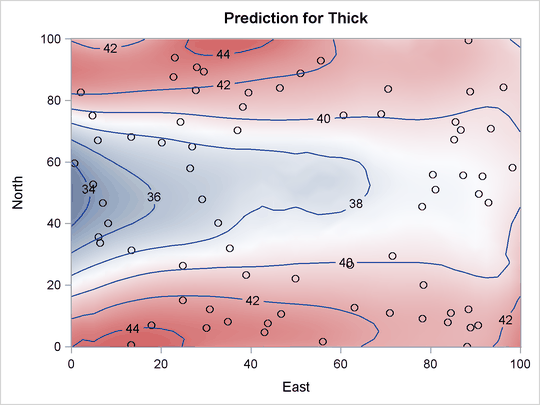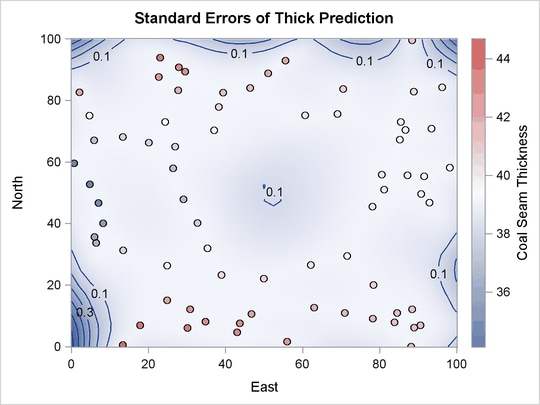### Contour Plots with PROC KRIGE2D

This example is taken from Example 49.2 of Chapter 49: The KRIGE2D Procedure. The coal seam thickness data set is available from the `Sashelp` library. The following statements create a SAS data set that contains a copy of these data along with some artificially added missing data:

```data thick;
set sashelp.thick;
if _n_ in (41, 42, 73) then thick = .;
run;
```

The following statements run PROC KRIGE2D:

```ods graphics on;

proc krige2d data=thick outest=predictions
plots=(observ(showmissing)
coordinates xc=East yc=North;
predict var=Thick r=60;
model scale=7.2881 range=30.6239 form=gauss;
grid x=0 to 100 by 2.5 y=0 to 100 by 2.5;
run;
```

The PLOTS=OBSERV(SHOWMISSING) option produces a scatter plot of the data along with the locations of any missing data. The PLOTS=PRED option produces maps of the kriging predictions and standard errors. Two instances of the PLOTS=PRED option are specified with suboptions that customize the plots. The results are shown in Figure 21.7.

Figure 21.7: Spatial Distribution